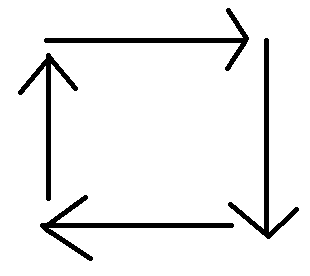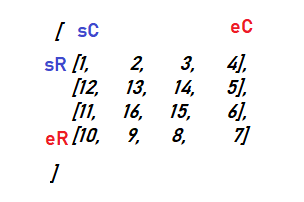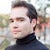# CoDEVIANT #21 (10/11/20) — Spiral MotionYou spin me right round, baby, right round like algorithm, baby right right round… XD
`[   [1,   2,  3, 4],   [12, 13, 14, 5],   [11, 16, 15, 6],   [10,  9,  8, 7]]`
• Read the top row from left to right, including the far right value.
• Read the far right column omitting the very top value and including the very bottom value.
• Read the bottom row from right to left omitting the right-most value and including the left-most value.
• Rad the far left column, omitting the far left bottom value and the far left top value.
`function spiralTraverse(array) {  // Write your code here.  let results = [] let startCol = 0 let startRow = 0 //endRow signifies the start places of each row up and down the verticle side of the block let endRow = array.length - 1 //endCol signifies the start places of each column across the top of the block let endCol = array.length - 1  while(startCol <= endCol && startRow <= endRow) {  //top  for(let col = startCol; col <= endCol ; col++) {   results.push(array[startRow][col])   console.log('top', results)  }    //right  for(let row = startRow + 1; row <= endRow; row++) {   results.push(array[row][endCol])   console.log('right', results)  }    //bottom  for(let column = endCol - 1; column >= startCol; column --) {   if(startRow === endRow) break   results.push(array[endRow][column])   console.log('bottom', results)  }    //left  for(let row = endRow - 1; row > startRow; row --) {   if(startCol === endCol) break   results.push(array[row][startCol])   console.log(results)  }    startCol++  startRow++  endCol--  endRow-- } return results}`
`let results = [] let startCol = 0 let startRow = 0 //endRow signifies the start places of each row up and down the verticle side of the block let endRow = array.length - 1 //endCol signifies the start places of each column across the top of the block let endCol = array.length - 1`startColumn (sC) is 0 to start. startRow (sR) is 0 to start. endColumn (eC) is the length of the first array in the 2D array “1,2,3,4” minus one -3-. endRow (eR) is the length of the 2D array “1,12,11,10”. The value is 3 as well.
`while(startCol <= endCol && startRow <= endRow) {   ...   startCol++   startRow++   endCol--   endRow--}`
• top // left to right
• right //top to bottom
• bottom // left to right
• left //bottom to top
`for(let col = startCol; col <= endCol; col++) {   results.push(array[startRow][col])  }`
`for(let row = startRow + 1; row <= endRow; row++) {   results.push(array[row][endCol])}`
`for(let column = endCol - 1; column >= startCol; column --) {   if(startRow === endRow) break   results.push(array[endRow][column])   console.log('bottom', results)  }`
`for(let row = endRow - 1; row > startRow; row --) {   if(startCol === endCol) break   results.push(array[row][startCol])   console.log(results)  }`

--

--

--

is a web developer, opera singer, actor, and lover of cats. (adrian-rosales.tech)

Love podcasts or audiobooks? Learn on the go with our new app.

## Working with JSON data in BigQuery## Deep dive to the dark side of React Context## GraphQL Server: What’s After ‘Hello, World’## App.js Conf 2020 canceled## Super Simple React/Redux Apps with Reduxigen: Step by Step## Writing versionable, releasable Vuejs components for use in any JS application## Understanding The Set Object in JavaScript## Why we need Redux?